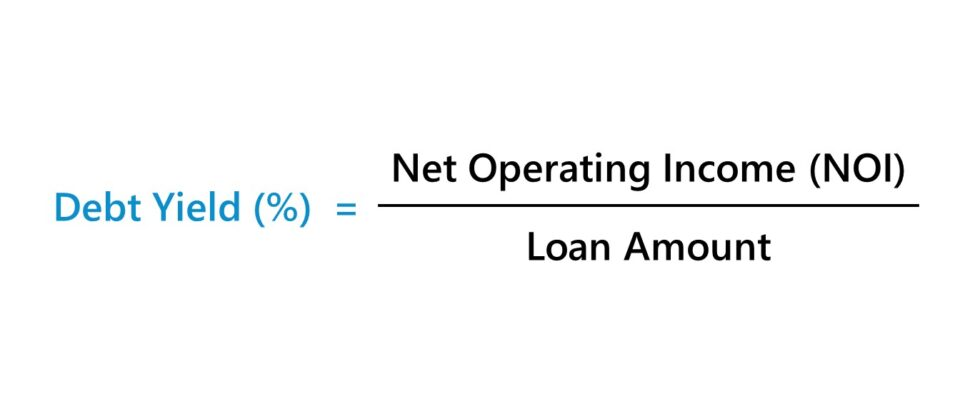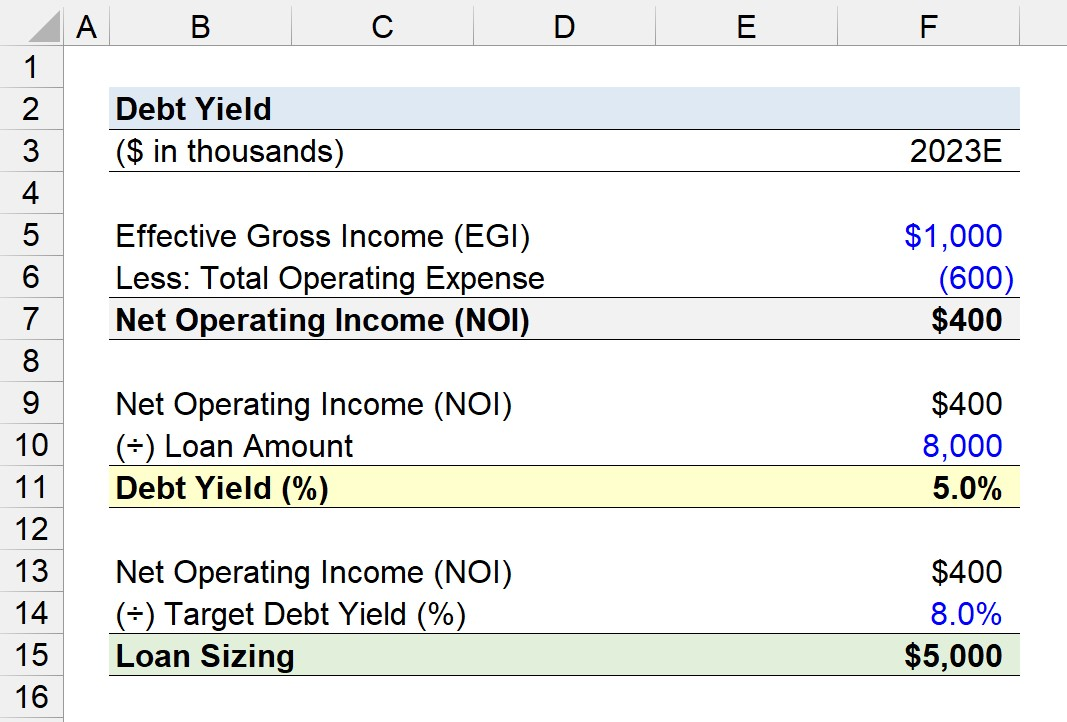Welcome to Wall Street Prep! Use code at checkout for 15% off.# Debt Yield

Guide to Understanding Debt Yield (DY)## How to Calculate Debt Yield?

The debt yield is frequently utilized by lenders in the commercial real estate market (CRE) as a method to measure credit risk in underwriting.

In short, the debt yield is the ratio between a property’s net operating income (NOI) and total loan amount, expressed as a percentage.

• Net Operating Income (NOI) → Net operating income, or “NOI” for short, is a fundamental real estate metric used to measure a property’s cash flows and profitability. The NOI of a rental property is calculated by first determining the property’s total income – the sum of its rental and ancillary income – and then subtracting its operating expenses.
• Loan Amount → The total loan amount refers to the outstanding principal balance associated with real estate financing, such as a mortgage loan.

Conceptually, the debt yield is the estimated return a real estate lender receives relative to the original loan amount provided to the borrower under the hypothetical scenario of default (and foreclosure).

Because non-operating costs such as financing costs and income taxes are not part of the net operating income (NOI) calculation, the metric is considered an unlevered, pre-tax measure of profitability (i.e. “capital structure neutral”). The debt yield is therefore suited for comparability purposes, akin to the EBITDA metric.

The items excluded from the NOI calculation, such as capital reserves, capital expenditures, and tenant improvements, are unrelated to the property’s core operations. Likewise, the debt service component is also excluded since it falls under the category of financing costs.

The process of computing the debt yield metric is a three-step process:

1. Calculate Net Operating Income (NOI)
2. Divide Net Operating Income (NOI) by the Loan Amount
3. Convert Debt Yield from Decimal to Percentage Form (Multiply by 100)## Debt Yield Formula

The formula to calculate the debt yield divides the net operating income (NOI) by the total loan amount.

Debt Yield (%) = Net Operating Income (NOI) ÷ Loan Amount

Where:

Net Operating Income (NOI) = (Total Rental Income + Ancillary Income) Total Operating Expenses
• Ancillary Income → The non-rental sources of income, such as reimbursements on utilities.
• Total Operating Expense → The costs tied to the operations of the property, such as repair and maintenance costs, marketing, general and administrative expenses, and utilities.

The debt yield formula can also be expressed as the property’s annual NOI divided by the difference between the purchase price and the down payment.

Debt Yield (%) = Net Operating Income (NOI) ÷ (Purchase Price – Down Payment)

Note: While income taxes are excluded in the net operating income (NOI) calculation, the property taxes paid by the owner must be included.

## What is a Good Debt Yield?

In practice, real estate lenders that provide commercial and multifamily loans use the debt yield metric to estimate the time required to recoup their initial investment, in the scenario where the property is seized post-default and now belongs to them.

While each lender sets different targets for the debt yield, the standard range among commercial real estate lenders (CMBS) is from around 8% to 12%.

The general rules of thumb for interpreting the debt yield are as follows:

• Lower Debt Yield → If the debt yield is low, the implied risk to the lender is higher, because the property’s operating cash flows could not meet the mandatory debt service.
• Higher Debt Yield → In contrast, the higher the debt yield, the less risk the financing poses due to the reduced likelihood of the borrower defaulting on the obligation.

But of course, most lenders tend to be risk-averse and prioritize the retrieval of the original principal at maturity. Therefore, measuring the potential downside risk in the event of foreclosure is part of worst-case scenario contingency planning.

Most institutional real estate lenders will set internal targets on the minimum debt yield in their underwriting process, wherein a borrowing unable to meet the minimum target will either be adjusted downward or declined.

Therefore, the more practical use-case of the debt yield metric pertains more toward debt sizing, i.e. quantifying the maximum amount of debt capital to put at risk based on the perceived riskiness of the borrowing.

However, the target yield is lender-specific and conditional on external factors, such as the current conditions of the credit markets, among other considerations.

###### Debt Yield vs. DSCR vs. Loan-to-Value Ratio (LTV)

There are two other risk measures of particular importance in real estate lending:

• Debt Service Coverage Ratio (DCSR)
• Loan-to-Value Ratio (LTV)

The debt yield is unique, however, because the metric is independent of fluctuating interest rates and real estate market values and unaffected by the borrowing term, i.e. length of the loan amortization schedule.

## Debt Yield Calculator

We’ll now move on to a modeling exercise, which you can access by filling out the form below.#### Excel TemplateSubmitting...

## 1. Commercial Real Estate Loan Assumptions (CRE)

Suppose a real estate lender is performing diligence on a potential borrower that has requested \$8 million in financing to purchase a property.

Once purchased, the property is expected to generate \$1 million in effective gross income (EGI) while incurring \$600k in total operating expenses.

By subtracting the total operating expense from the effective gross income (EGI), the property’s net operating income (NOI) comes out to \$400k.

• Net Operating Income (NOI) = \$1 million – \$600k = \$400k

## 2. Debt Yield Calculation Example

In the next step, we’ll calculate the debt yield on the proposed financing arrangement using the two inputs from the prior section.

The implied debt yield in our financing scenario is 5.0%, which we determined by dividing the property’s net operating income (NOI) by the requested loan amount from the borrower.

• Debt Yield (%) = \$400k ÷ \$8 million = 5.0%

To reiterate from earlier, lenders prefer higher debt yields to limit the downside risk and potential for incurring losses.

Therefore, the 5.0% debt yield will most likely result in the lender declining the requested loan unless the terms of the financing are adjusted, i.e. via a reduction in the size of the loan.

## 3. Debt Yield Loan Sizing Analysis Example

The final section of our debt yield modeling exercise will consist of loan sizing, where we’ll rearrange the formula from earlier to determine the maximum loan amount the lender should offer to meet its target yield.

Loan Sizing = Net Operating Income (NOI) ÷ Target Debt Yield (%)

The minimum target yield of the lender is assumed to be 8.0% here.

• Target Debt Yield (%) = 8.0%

In closing, we arrive at \$5 million as the maximum loan amount upon dividing the borrower’s net operating income (NOI) by the target debt yield.

• Loan Sizing = \$400k ÷ 8.0% = \$5 million20+ Hours of Online Video Training

### Master Real Estate Financial Modeling

This program breaks down everything you need to build and interpret real estate finance models. Used at the world's leading real estate private equity firms and academic institutions.

Inline Feedbacks